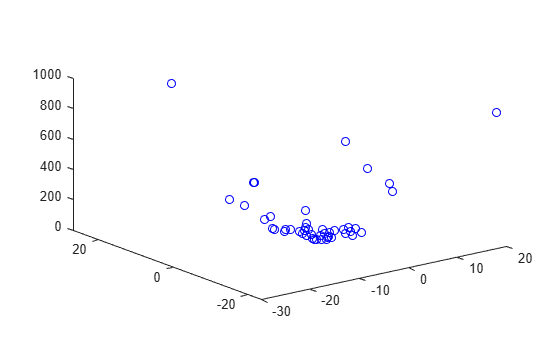# Find Multiple Local Solutions Using `MultiStart` or `GlobalSearch`, Problem-Based

In solutions to problems that use the `MultiStart` or `GlobalSearch` function with the problem-based approach, the `output` structure contains a field named `local` that has information about the local solutions. For example, find the local solutions to the `rastriginsfcn` function of two variables, which is available when you run this example, by using `MultiStart`.

```x = optimvar("x",LowerBound=-50,UpperBound=50); y = optimvar("y",LowerBound=-50,UpperBound=50); fun = rastriginsfcn([x,y]); prob = optimproblem(Objective=fun); ms = MultiStart; x0.x = -30; x0.y = 20; rng default % For reproducibility [sol,fval,exitflag,output] = solve(prob,x0,ms,MinNumStartPoints=50);```
```Solving problem using MultiStart. MultiStart completed the runs from all start points. All 50 local solver runs converged with a positive local solver exitflag. ```
`disp(sol)`
``` x: 6.8842e-10 y: 1.0077e-09 ```
`disp(fval)`
``` 0 ```

How many local solutions does `MultiStart` find?

```multisols = output.local.sol; N = numel(multisols)```
```N = 46 ```

Plot the values.

`plot3(multisols.x,multisols.y,multisols.Objective,'bo')``MultiStart` starts from 50 initial points, but finds only 46 solutions. `MultiStart` reports that all runs converged. Therefore, some solutions have multiple initial points leading to those solutions. Find the `x0` values that list multiple initial points.

```myx0 = output.local.x0; sx = zeros(size(myx0)); for i = 1:length(sx) sx(i) = numel(myx0{i}); end mults = find(sx >= 2)```
```mults = 1×4 13 17 25 36 ```

Determine whether `fmincon`, starting from two initial points in `mults(1)`, ends at the same solution.

```pts = myx0(mults(1)); r = pts{1}.x; t01.x = r(1); s = pts{1}.y; t01.y = s(1); disp(t01)```
``` x: -30 y: 20 ```
```opts = optimoptions("fmincon",Display="none"); sol1 = solve(prob,t01,Options=opts)```
```sol1 = struct with fields: x: -1.9899 y: -2.9849 ```
```t02.x = r(2); t02.y = s(2); disp(t02)```
``` x: 34.9129 y: -24.5718 ```
`sol2 = solve(prob,t02,Options=opts)`
```sol2 = struct with fields: x: -1.9899 y: -2.9849 ```

Even though the starting points `t01` and `t02` are far apart, the solutions `sol1` and `sol2` are identical to display precision.